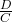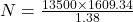## Determine the number of revolutions through which a typical automobile tire turns in 1 yr. Suppose the automobile travels 13500 miles each y

Question

Determine the number of revolutions through which a typical automobile tire turns in 1 yr. Suppose the automobile travels 13500 miles each year on tires with radius 0.220 m.

in progress 0
4 months 2021-09-04T22:32:48+00:00 1 Answers 6 views 0

Number of revolution made by tire is 1.57 x 10⁷

Explanation:

Radius of tire, r = 0.220 m

Circumference of tire, C = 2πr

Substitute the value of r in the above equation.

C = 2 x π x 0.220 m = 1.38 m

Total distance covered by tire in a year, D = 13500 miles

But 1 mile = 1609.34 m

So, D = 13500 x 1609.34 m

Number of revolutions take by tire, N =N = 15743543